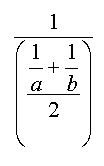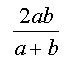## Saturday, 22 November 2008

### Harmony, and Harmonic Problems

Things happen in threes according to the old myth, and in this case it was true. I was doing some research on the early history of a mathematical problem often called the "cistern" problem. You probably know the type; "If one pipe can fill a cistern in 6 hours and another can fill it in four hours, how long would it take both pipes working together." While I was working on that, I got a nice article sent to me on the first proof that the harmonic sequence diverges... and then, I was reading a blog by Dave Marain Math Notationsin which he posed a problem that asked, in its general form, given a square inscribed in a right triangle (with one corner at the right angle of the triangle), what is the length of a side of the square in terms of the legs of the triangle.

So what do all these have in common with each other. dare I say what makes them in "harmony"?.... the answer is Harmony, or at least the mathematical relationship of the harmonic mean.

To the early Greeks, if Nichomachus can be believed, all the means were descriptive of musical relations. Much is often made of the Harmonic Mean in relation to a musical sense, but this may not represent the Greek view. Euclid used the word enarmozein to describe a segment that just fits in a given circle. The word is a form of the word Harmozein which the more competent Greek Scholars tell me means to join or to fit together. Jeff Miller's Web site on the first use of Mathematical terms contains a reference to the very early origin of the harmonic mean, 'A surviving fragment of the work of Archytas of Tarentum (ca. 350 BC) states, 'There are three means in music: one is the arithmetic, the second is the geometric, and the third is the subcontrary, which they call harmonic.' The term harmonic mean was also used by Aristotle. "
My search for the early roots of the cistern problem had taken me back to Heron's Metre'seis around the year fifty of the common era. The problem became a staple in arithmetics and problem books and was used by Alcuin (775) and appears in the Lilavati of Bhaskara (1150). I found the illustration I used on the blog for The First Illustrated Arithmetic a few days ago, from the 1492 arithmetic, Trattato di aritmetica by Filippo Calandri.
The solution to a cistern problem is the harmonic mean of the times taken by each pipe. For example, one problem asks "If one pipe can fill a cistern in three hours, and a second can fill it in five hours, how fast will the two pipes take to fill the cistern if both are opened at once. The solution is given by finding the average rate of fill of the two rates, the harmonic mean of three and five, which is three and three-quarter hours. But as the name "mean" suggest, that's the average rate of the two so working together, they would take one-half the time, one and seven-eighths hours, or about an hour and 53 minutes.

The Harmonic mean is the reciprocal of the mean of the reciprocals of the values, so for values a and b, the harmonic mean is given bywhich for two numbers can be simplified to the more economicalHeron might have been the first recorded example of a cistern problem, but a problem calling on the reader to use the harmonic mean occurs even earlier in the Rhind Mathematical Papyrus, now located in the British Museum, in problem 76. The problem involves making loaves of bread with different qualities, but the solution is still the harmonic mean. (I have learned from David Singmaster's Chronology of Recreational Mathematics that the cistern problem appeared, perhaps 300 years before Heron's use, in China by Chiu Chang Suan Shu (around 150 BC).

The series of terms formed by the reciprocals of the positive integers is a common torment for college students in their first introduction to analysis. The sequencein which each number gets smaller and smaller seems to very slowly approach some upper limit. Even after adding 250,000,000 terms, the sum is still less than twenty, and yet... in the mid 1300's, Nichole d'Oresme showed that it will eventually pass any value you can name. In short, it diverges, slowly, very, very slowly, to infinity. Even when warned, it seems like students want to believe it converges. A well-known anecdote about a teacher trying to get student's to remember that it diverges goes:
"Today I said to the calculus students, “I know, you’re looking at this series and you don’t see what I’m warning you about. You look and it and you think, ‘I trust this series. I would take candy from this series. I would get in a car with this series.’ But I’m going to warn you, this series is out to get you. Always remember: The harmonic series diverges. Never forget it.”<\blockquote>

By the way, each number in the harmonic series is the harmonic mean of the numbers on each side of it (so 1/2 and 1/4 have a harmonic mean of 1/3), and in fact, of any numbers equally spaced away from it such as 1 and 1/5 also have a harmonic mean of 1/3.

And then, I came across that little problem of a square inscribed in a right triangle. If the two legs are a and b, then the sides of the square will have a length equal to the one-half the harmonic mean of a and b .  More generally, a square inscribe in any triangle with one side along a base will have sides equal to one half the harmonic mean of the base and the altitude to that base.  There are lots of other interesting problems that yeild to the use of the harmonic mean, and I mean to write again on that collection.

So I guess things do come in threes, unless I come across another one, but whether it comes in threes or fours, it all seems to work together, in perfect harmony.

Dave Marain said...

Pat, we share a passion for the history of mathematics and how seemingly unrelated ideas are interconnected. I didn't expect the harmonic mean to appear in my square in the triangle problem. Yet somehow I wasn't that surprised when it did!

Thanks for the reference and I enjoyed reading your post. BTW, I haven't participated or even looked at math-learn, math-teach or those other forums to which I used to post, but it was nice to find your blog and see your name again after all these years...
Dave Marain

Pat B said...

Thanks Dave; and I should add that I failed to mention many variations of the problem that problem solvers may encounter across history. In the 1400's, Smith mentions, the animals eating a goat version (if a lion can eat a goat in three days and a tiger in five days...). Later, in the mid 1500's, problems involving A building a house in five days and B building a house in ten days, appeared. Today it is most often seen, it seems, in questions about driving from here to there and back at different speeds. In each case, the result leads to the Harmonic mean.Dennis said...

Pat: In your cistern example, how can the answer be three and three-quarters? That's more time than the first pipe requires alone. Wouldn't the answer be half of the harmonic mean?

Then in the problem of the inscribed square in a right triangle, isn't the length of each side of the square half the harmonic mean?

Dennis

Pat B said...

Dennis, and others, it is half the harmonic mean (since we combine two things working at the "average" speed.